# vehicleCostmap

Costmap representing planning space around vehicle

## Description

The `vehicleCostmap` object creates a costmap that represents the planning search space around a vehicle. The costmap holds information about the environment, such as obstacles or areas that the vehicle cannot traverse. To check for collisions, the costmap inflates obstacles using the inflation radius specified in the `CollisionChecker` property. The costmap is used by path planning algorithms, such as `pathPlannerRRT`, to find collision-free paths for the vehicle to follow.

The costmap is stored as a 2-D grid of cells, often called an occupancy grid or occupancy map. Each grid cell in the costmap has a value in the range [0, 1] representing the cost of navigating through that grid cell. The state of each grid cell is free, occupied, or unknown, as determined by the `FreeThreshold` and `OccupiedThreshold` properties.

The following figure shows a costmap with sample costs and grid cell states.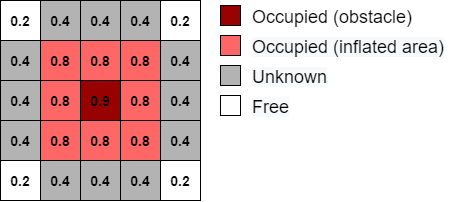## Creation

### Syntax

``costmap = vehicleCostmap(C)``
``costmap = vehicleCostmap(mapWidth,mapLength)``
``costmap = vehicleCostmap(mapWidth,mapLength,costVal)``
``costmap = vehicleCostmap(occMap)``
``costmap = vehicleCostmap(___,'MapLocation',mapLocation)``
``costmap = vehicleCostmap(___,Name,Value)``

### Description

````costmap = vehicleCostmap(C)` creates a vehicle costmap using the cost values in matrix `C`.```
````costmap = vehicleCostmap(mapWidth,mapLength)` creates a vehicle costmap representing an area of width `mapWidth` and length `mapLength` in world units. By default, each grid cell is in the unknown state.```

example

````costmap = vehicleCostmap(mapWidth,mapLength,costVal)` also assigns a default cost, `costVal`, to each cell in the grid.```
````costmap = vehicleCostmap(occMap)` creates a vehicle costmap from the occupancy map `occMap`. Use of this syntax requires Navigation Toolbox™.```
````costmap = vehicleCostmap(___,'MapLocation',mapLocation)` specifies in `mapLocation` the bottom-left corner coordinates of the costmap. Specify `'MapLocation',mapLocation` after any of the preceding inputs and in any order among the `Name,Value` pair arguments. ```
````costmap = vehicleCostmap(___,Name,Value)` uses `Name,Value` pair arguments to specify the `FreeThreshold`, `OccupiedThreshold`, `CollisionChecker`, and `CellSize` properties. For example, `vehicleCostmap(C,'CollisionChecker',ccConfig)` uses an `inflationCollisionChecker` object, `ccConfig`, to represent the vehicle shape and check for collisions. After you create the object, you can update all of these properties except `CellSize`.```

### Input Arguments

expand all

Cost values, specified as a matrix of real values that are in the range [0, 1].

When creating a `vehicleCostmap` object, if you do not specify `C` or a uniform cost value, `costVal`, then the default cost value of each grid cell is `(FreeThreshold + OccupiedThreshold)/2`.

Data Types: `single` | `double`

Width of costmap, in world units, specified as a positive real scalar.

Length of costmap, in world units, specified as a positive real scalar.

Uniform cost value applied to all cells in the costmap, specified as a real scalar in the range [0, 1].

When creating a `vehicleCostmap` object, if you do not specify `costVal` or a cost value matrix, `C`, then the default cost value of each grid cell is `(FreeThreshold + OccupiedThreshold)/2`.

Occupancy map, specified as an `occupancyMap` (Navigation Toolbox) or `binaryOccupancyMap` (Navigation Toolbox) object. Use of this argument requires Navigation Toolbox.

Costmap location, specified as a two-element real-valued vector of the form [mapX mapY]. This vector specifies the coordinate location of the bottom-left corner of the costmap.

Example: `'MapLocation',[8 8]`

## Properties

expand all

Threshold below which a grid cell is free, specified as a real scalar in the range [0, 1].

A grid cell with cost c can have one of these states:

• If c < `FreeThreshold`, the grid cell state is free.

• If c`FreeThreshold` and c`OccupiedThreshold`, the grid cell state is unknown.

• If c > `OccupiedThreshold`, the grid cell state is occupied.

Threshold above which a grid cell is occupied, specified as a real scalar in the range [0, 1].

A grid cell with cost c can have one of these states:

• If c < `FreeThreshold`, the grid cell state is free.

• If c`FreeThreshold` and c`OccupiedThreshold`, the grid cell state is unknown.

• If c > `OccupiedThreshold`, the grid cell state is occupied.

Collision-checking configuration, specified as an `InflationCollisionChecker` object. To create this object, use the `inflationCollisionChecker` function. Using the properties of the `InflationCollisionChecker` object, you can configure:

• The inflation radius used to inflate obstacles in the costmap

• The number of circles used to enclose the vehicle when calculating the inflation radius

• The placement of each circle along the longitudinal axis of the vehicle

• The dimensions of the vehicle

By default, `CollisionChecker` uses the default `InflationCollisionChecker` object, which is created using the syntax `inflationCollisionChecker()`. This collision-checking configuration encloses the vehicle in one circle.

Extent of costmap around the vehicle, specified as a four-element, nonnegative integer vector of the form [xmin xmax ymin ymax].

• xmin and xmax describe the length of the map in world coordinates.

• ymin and ymax describe the width of the map in world coordinates.

Side length of each square cell, in world units, specified as a positive real scalar. For example, a side length of `1` implies a grid where each cell is a square of size 1-by-1 meters. Smaller values improve the resolution of the search space at the cost of increased memory consumption.

You can specify `CellSize` when you create the `vehicleCostmap` object. However, after you create the object, `CellSize` becomes read-only.

Size of costmap grid, specified as a two-element, positive integer vector of the form [nrows ncols].

• nrows is the number of grid cell rows in the costmap.

• ncols is the number of grid cell columns in the costmap.

## Object Functions

 `checkFree` Check vehicle costmap for collision-free poses or points `checkOccupied` Check vehicle costmap for occupied poses or points `getCosts` Get cost value of cells in vehicle costmap `setCosts` Set cost value of cells in vehicle costmap `plot` Plot vehicle costmap

## Examples

collapse all

Create a 10-by-20 meter costmap that is divided into square cells of size 0.5-by-0.5 meters. Specify a default cost value of 0.5 for all cells.

```mapWidth = 10; mapLength = 20; costVal = 0.5; cellSize = 0.5; costmap = vehicleCostmap(mapWidth,mapLength,costVal,'CellSize',cellSize)```
```costmap = vehicleCostmap with properties: FreeThreshold: 0.2000 OccupiedThreshold: 0.6500 CollisionChecker: [1x1 driving.costmap.InflationCollisionChecker] CellSize: 0.5000 MapSize: [40 20] MapExtent: [0 10 0 20] ```

Mark an obstacle on the costmap. Display the costmap.

```occupiedVal = 0.9; xyPoint = [2,4]; setCosts(costmap,xyPoint,occupiedVal) plot(costmap)```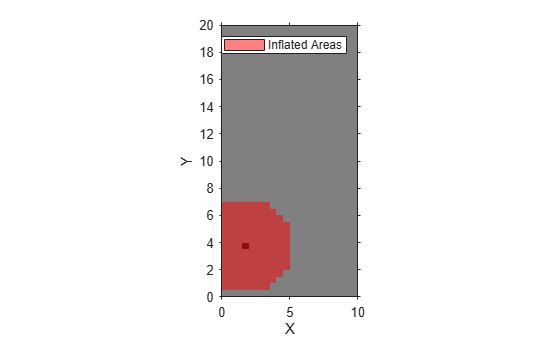Mark an obstacle-free area on the costmap. Display the costmap again.

```freeVal = 0.15; [X,Y] = meshgrid(3.5:cellSize:5,0.5:cellSize:1.5); setCosts(costmap,[X(:),Y(:)],freeVal) plot(costmap) ```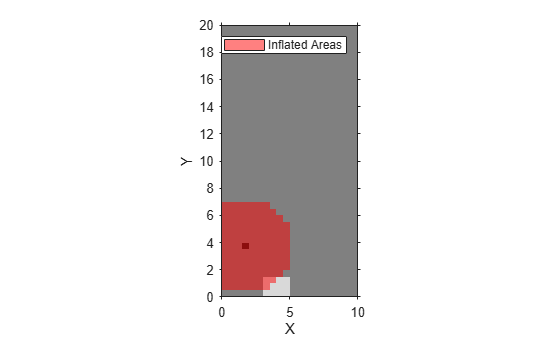## Algorithms

To simplify checking for whether a vehicle pose is in collision, `vehicleCostmap` inflates the size of obstacles. The collision-checking algorithm follows these steps:

1. Calculate the inflation radius, in world units, from the vehicle dimensions. The default inflation radius is equal to the radius of the smallest set of overlapping circles required to completely enclose the vehicle. The center points of the circles lie along the longitudinal axis of the vehicle. Increasing the number of circles decreases the inflation radius, which enables more precise collision checking.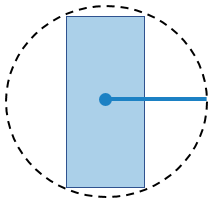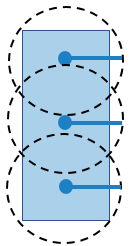2. Convert the inflation radius to a number of grid cells, R. Round up noninteger values of R to the next largest integer.

3. Inflate the size of obstacles using R. Label all cells in the inflated area as occupied.

The diagrams show occupied cells in dark red. Cells in the inflated area are colored in light red. The solid black line shows the original inflation radius. In the diagram on the left, R is 3. In the diagram on the right, R is 2.

Inflated Grid Cells, One CenterInflated Grid Cells, Three Centers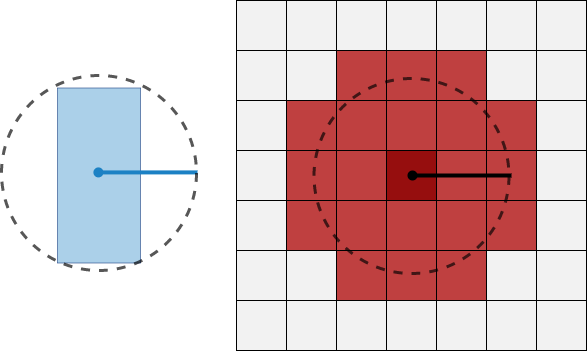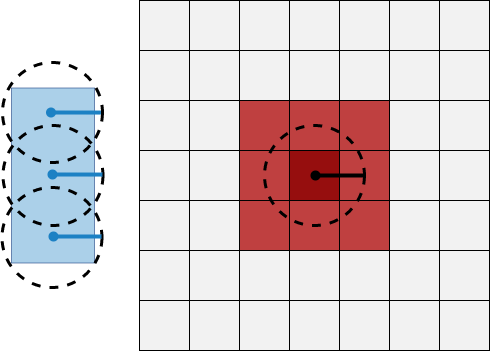4. Check whether the center points of the vehicle lie on inflated grid cells.

• If any center point lies on an inflated grid cell, then the vehicle pose is occupied. The `checkOccupied` function returns `true`. An occupied pose does not necessarily mean a collision. For example, the vehicle might lie on an inflated grid cell but not on the grid cell that is actually occupied.

• If no center points lie on inflated grid cells, and the cost value of each cell containing a center point is less than `FreeThreshold`, then the vehicle pose is free. The `checkFree` function returns `true`.

• If no center points lie on inflated grid cells, and the cost value of any cell containing a center point is greater than `FreeThreshold`, then the vehicle pose is unknown. Both `checkFree` and `checkOccupied` return `false`.

The following poses are considered in collision because at least one center point is on an inflated area.

Pose in Collision, One CenterPose in Collision, Three Centers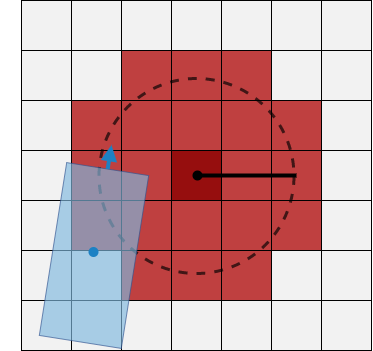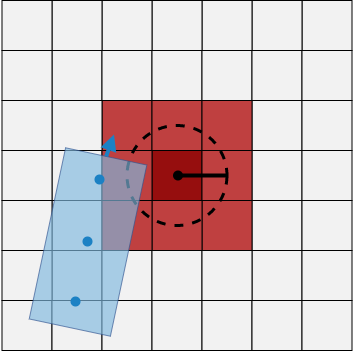## Compatibility Considerations

expand all

Errors starting in R2020b